Courses

# Use number line and add the following integers - Class 6 Class 6 Notes | EduRev

## Class 6 : Use number line and add the following integers - Class 6 Class 6 Notes | EduRev

The document Use number line and add the following integers - Class 6 Class 6 Notes | EduRev is a part of Class 6 category.
All you need of Class 6 at this link: Class 6

Use number line and add the following integers

Use number line and add the following integers:
(a) 9 + (− 6)
(b) 5 + (− 11)
(c) (− 1) + (− 7)
(d) (− 5) + 10
(e) (− 1) + (− 2) + (− 3)
(f) (− 2) + 8 + (− 4)
Two important things to remember
When we add positive integer, we move to the right
When we add negative integer, we move to the right

a) 9 + (− 6)
We start with number 9 and move to left six steps and we reach the number 3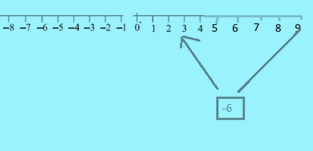b) 5 + (− 11)
We start with number 5 and move to left 11 steps and we reach the number -6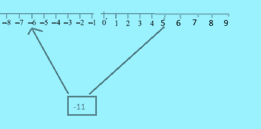c) (− 1) + (− 7)
We start with number -1 and move to left 7 steps and we reach the number -8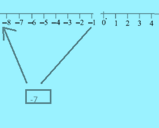d) (− 5) + 10
We start with number -5 and move to right 10 steps and we reach the number 5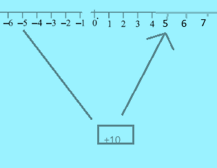e) (− 1) + (− 2) + (− 3)
We start with number -1 and move to left 2 steps and we reach the number -3. Then again we move to left 3 steps and we reach finally -6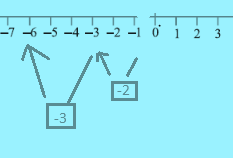f) (− 2) + 8 + (− 4)
We start with number -2 and move to right 8 steps and we reach the number 6. Then again we move to left 4 steps and we reach finally 2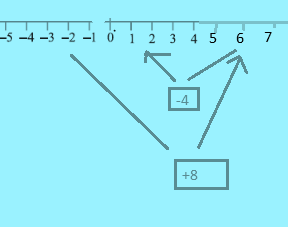Offer running on EduRev: Apply code STAYHOME200 to get INR 200 off on our premium plan EduRev Infinity!

,

,

,

,

,

,

,

,

,

,

,

,

,

,

,

,

,

,

,

,

,

;